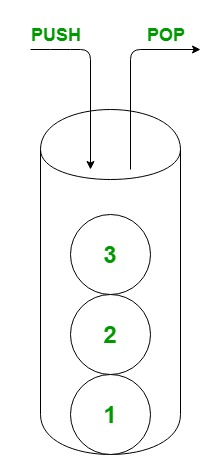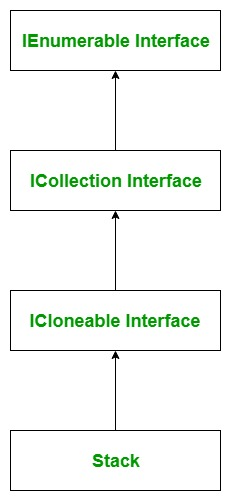# C# Stack with Examples

A Stack represents a last-in, first-out collection of objects. It is used when you need last-in, first-out access to items. It is both a generic and non-generic type of collection. The generic stack is defined in System.Collections.Generic namespace whereas non-generic stack is defined under System.Collections namespace, here we will discuss non-generic type stack. A stack is used to create a dynamic collection that grows, according to the need of your program. In a stack, you can store elements of the same type or different types.The below diagram illustrates the Stack class hierarchy:Important Points:

• The Stack class implements the IEnumerable, ICollection, and ICloneable interfaces.
• When you add an item in the list, it is called pushing the element.
• When you remove it, it is called popping the element.
• The capacity of a Stack is the number of elements the Stack can hold. As elements are added to a Stack, the capacity is automatically increased as required through reallocation.
• In Stack, you are allowed to store duplicate elements.
• A Stack accepts null as a valid value for reference types.

#### How to create a Stack?

Stack class has three constructors which are used to create a stack which is as follows:

• Stack(): This constructor is used to create an instance of the Stack class which is empty and having the default initial capacity.
• Stack(ICollection): This constructor is used to create an instance of the Stack class which contains elements copied from the specified collection, and has the same initial capacity as the number of elements copied.
• Stack(Int32): This constructor is used to create an instance of the Stack class which is empty and having specified initial capacity or the default initial capacity, whichever is greater.

Let’s see how to create a stack using Stack() constructor:
Step 1: Include System.Collections namespace in your program with the help of using keyword.

`using System.Collections;`

Step 2: Create a stack using Stack class as shown below:

`Stack stack_name = new Stack();`

Step 3: If you want to add elements in your stack, then use Push() method to add elements in your stack. As shown in the below example.

Example:

## C#

 `// C# program to illustrate how to` `// create a stack` `using` `System;` `using` `System.Collections;`   `class` `GFG {`   `    ``// Main Method` `    ``static` `public` `void` `Main()` `    ``{`   `        ``// Create a stack` `        ``// Using Stack class` `        ``Stack my_stack = ``new` `Stack();`   `        ``// Adding elements in the Stack` `        ``// Using Push method` `        ``my_stack.Push(``"Geeks"``);` `        ``my_stack.Push(``"geeksforgeeks"``);` `        ``my_stack.Push(``'G'``);` `        ``my_stack.Push(``null``);` `        ``my_stack.Push(1234);` `        ``my_stack.Push(490.98);`   `        ``// Accessing the elements` `        ``// of my_stack Stack` `        ``// Using foreach loop` `        ``foreach``(``var` `elem ``in` `my_stack)` `        ``{` `            ``Console.WriteLine(elem);` `        ``}` `    ``}` `}`

Output:

```490.98
1234

G
geeksforgeeks
Geeks```

#### How to remove elements from the Stack?

In Stack, you are allowed to remove elements from the stack. The Stack class provides two different methods to remove elements and the methods are:

• Clear: This method is used to remove all the objects from the stack.
• Pop: This method removes the beginning element of the stack.

Example:

## C#

 `// C# program to illustrate how to` `// remove elements from the stack` `using` `System;` `using` `System.Collections;`   `class` `GFG {`   `    ``// Main Method` `    ``static` `public` `void` `Main()` `    ``{`   `        ``// Create a stack` `        ``// Using Stack class` `        ``Stack my_stack = ``new` `Stack();`   `        ``// Adding elements in the Stack` `        ``// Using Push method` `        ``my_stack.Push(``"Geeks"``);` `        ``my_stack.Push(``"geeksforgeeks"``);` `        ``my_stack.Push(``"geeks23"``);` `        ``my_stack.Push(``"GeeksforGeeks"``);`   `        ``Console.WriteLine(``"Total elements present in"``+` `                    ``" my_stack: {0}"``, my_stack.Count);` `                                                    `  `        ``my_stack.Pop();`   `        ``// After Pop method` `        ``Console.WriteLine(``"Total elements present in "``+` `                      ``"my_stack: {0}"``, my_stack.Count);`   `                                                   `  `        ``// Remove all the elements` `        ``// from the stack` `        ``my_stack.Clear();`   `        ``// After Pop method` `        ``Console.WriteLine(``"Total elements present in "``+` `                      ``"my_stack: {0}"``, my_stack.Count);` `                                                   `  `    ``}` `}`

Output:

```Total elements present in my_stack: 4
Total elements present in my_stack: 3
Total elements present in my_stack: 0```

#### How to get the topmost element of the Stack?

In Stack, you can easily find the topmost element of the stack by using the following methods provided by the Stack class:

• Pop: This method returns the object at the beginning of the stack with modification means this method removes the topmost element of the stack.
• Peek: This method returns the object at the beginning of the stack without removing it.

Example:

## C#

 `// C# program to illustrate how to` `// get topmost elements of the stack` `using` `System;` `using` `System.Collections;`   `class` `GFG {`   `    ``// Main Method` `    ``static` `public` `void` `Main()` `    ``{`   `        ``// Create a stack` `        ``// Using Stack class` `        ``Stack my_stack = ``new` `Stack();`   `        ``// Adding elements in the Stack` `        ``// Using Push method` `        ``my_stack.Push(``"Geeks"``);` `        ``my_stack.Push(``"geeksforgeeks"``);` `        ``my_stack.Push(``"geeks23"``);` `        ``my_stack.Push(``"GeeksforGeeks"``);`   `        ``Console.WriteLine(``"Total elements present in"``+` `                     ``" my_stack: {0}"``,my_stack.Count);` `                                                   `  `        ``// Obtain the topmost element ` `        ``// of my_stack Using Pop method` `        ``Console.WriteLine(``"Topmost element of my_stack"` `                          ``+ ``" is: {0}"``,my_stack.Pop());` `                          `  `        ``Console.WriteLine(``"Total elements present in"``+` `                    ``" my_stack: {0}"``, my_stack.Count);` `                         `  `        ``// Obtain the topmost element ` `        ``// of my_stack Using Peek method` `        ``Console.WriteLine(``"Topmost element of my_stack "``+` `                              ``"is: {0}"``,my_stack.Peek());` `                          `    `        ``Console.WriteLine(``"Total elements present "``+` `                 ``"in my_stack: {0}"``,my_stack.Count);` `                          `  `    ``}` `}`

Output:

```Total elements present in my_stack: 4
Topmost element of my_stack is: GeeksforGeeks
Total elements present in my_stack: 3
Topmost element of my_stack is: geeks23
Total elements present in my_stack: 3```

#### How to check the availability of elements in the stack?

In a stack, you can check whether the given element is present or not using Contains() method. Or in other words, if you want to search an element in the given stack use Contains() method. This method returns true if the element present in the stack. Otherwise, return false.

Note: The Contains() method takes O(n) time to check if the element exists in the stack. This should be taken into consideration while using this method.

Example:

## C#

 `// C# program to illustrate how` `// to check element present in` `// the stack or not` `using` `System;` `using` `System.Collections;`   `class` `GFG {`   `    ``// Main  Method` `    ``static` `public` `void` `Main()` `    ``{`   `        ``// Create a stack` `        ``// Using Stack class` `        ``Stack my_stack = ``new` `Stack();`   `        ``// Adding elements in the Stack` `        ``// Using Push method` `        ``my_stack.Push(``"Geeks"``);` `        ``my_stack.Push(``"geeksforgeeks"``);` `        ``my_stack.Push(``"geeks23"``);` `        ``my_stack.Push(``"GeeksforGeeks"``);`   `        ``// Checking if the element is` `        ``// present in the Stack or not` `        ``if` `(my_stack.Contains(``"GeeksforGeeks"``) == ``true``) ` `        ``{` `            ``Console.WriteLine(``"Element is found...!!"``);` `        ``}`   `        ``else` `        ``{` `            ``Console.WriteLine(``"Element is not found...!!"``);` `        ``}` `    ``}` `}`

Output:

`Element is found...!!`

#### Generic Stack Vs Non-Generic Stack

Whether you're preparing for your first job interview or aiming to upskill in this ever-evolving tech landscape, GeeksforGeeks Courses are your key to success. We provide top-quality content at affordable prices, all geared towards accelerating your growth in a time-bound manner. Join the millions we've already empowered, and we're here to do the same for you. Don't miss out - check it out now!

Previous
Next